#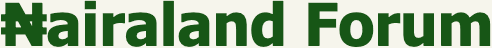Welcome, Guest: Join Nairaland / LOGIN! / Trending / Recent / New
Stats: 2,480,950 members, 5,616,054 topics. Date: Monday, 25 May 2020 at 12:27 AM

## If You Fail This Test, Then Stop Posting Here - Education (2) - Nairaland

(1) (2) (3) (4) (5) (6) (Reply) (Go Down)

 Re: If You Fail This Test, Then Stop Posting Here by APCLyingBastard: 9:56pm On May 23 GamalNasser:2/3 Re: If You Fail This Test, Then Stop Posting Here by APCLyingBastard: 9:57pm On May 23 Sammy07: I think the answer is 2/3, since the fact that you picked a Gold ball implies a 2x likelihood that you’re in the GG box than the GS box. The other way to look at this is to realize that the first pick has 6 distinct balls that you can pick. The fact that the balls are in 3 boxes doesn’t affect the 1/6 likelihood that you will pick any one of the 6 balls. Of course, the second pick is dependent on the first pick.Let’s say the balls are organized as such: [1, 2] [3, 4] [5, 6], or [G G] [G S] [S S]. You have picked one of balls 1, 2, 3. So, the possible second picks are 2, 1, 4 two of which are G and one is S.Tell us the answer, but don't ever say it's 50%Comprehension is your problem 1 Like Re: If You Fail This Test, Then Stop Posting Here by Kyrx(m): 9:58pm On May 23 The chance is 50% nah 3 Likes 1 Share Re: If You Fail This Test, Then Stop Posting Here by falcon01: 9:58pm On May 23 APCLyingBastard:IQ tests need to be mandatory to post here. So I am kicking it off with this very rudimentary test.Provide reasons for your answer.See pic below for test.2/5 1 Like Re: If You Fail This Test, Then Stop Posting Here by Kyrx(m): 9:59pm On May 23 APCLyingBastard:[s][/s]Comprehension is your problemBut you don't have to insult anyone to make your point/s. 3 Likes 1 Share Re: If You Fail This Test, Then Stop Posting Here by APCLyingBastard: 9:59pm On May 23 falcon01:2/5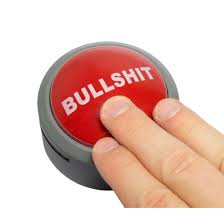Re: If You Fail This Test, Then Stop Posting Here by phelonrays: 10:01pm On May 23 Op thanks for racking up my brain this night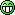Having evaluate the substance that lead to the appearing of the gold cum silver ball on the box, it relatively indicate and showcase the probability of picking the unseen gold ball at a proximity of 2:14 close meter rangeSicknesses is not good, sha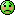Re: If You Fail This Test, Then Stop Posting Here by Kyrx(m): 10:01pm On May 23 Total number of balls = 6Total number of gold balls = 3Probability of picking a gold ball at random = 3÷6 =½ or 50% 1 Like Re: If You Fail This Test, Then Stop Posting Here by APCLyingBastard: 10:02pm On May 23 phelonrays:Op thanks for racking up my brain this nightHaving evaluate the substance that lead to the appearing of the gold cum silver ball on the box, it relatively indicate and showcase the probability of picking the unseen gold ball at a proximity of 2:14 close meter rangeSicknesses is not good, shaRe: If You Fail This Test, Then Stop Posting Here by APCLyingBastard: 10:03pm On May 23 Kyrx:Total number of balls = 6Total number of gold balls = 3Probability of picking a gold ball at random = 3÷6 =½ or 50%You are wrong in your reasons and as such you failed the test.Go back and read the question well and provide the right reason for your answer. 2 Likes Re: If You Fail This Test, Then Stop Posting Here by Sammy07: 10:04pm On May 23 APCLyingBastard:[s][/s]Comprehension is your problemThe problem can be reframed by describing the boxes as each having one drawer on each of two sides. Each drawer contains a coin. One box has a gold coin on each side (GG), one a silver coin on each side (SS), and the other a gold coin on one side and a silver coin on the other (GS). A box is chosen at random, a random drawer is opened, and a gold coin is found inside it. What is the chance of the coin on the other side being gold?The following reasoning appears to give a probability of 1/2:Originally, all three boxes were equally likely to be chosen.The chosen box cannot be box SS.So it must be box GG or GS.The two remaining possibilities are equally likely. So the probability that the box is GG, and the other coin is also gold, is 1/2.The flaw is in the last step. While those two cases were originally equally likely, the fact that you are certain to find a gold coin if you had chosen the GG box, but are only 50% sure of finding a gold coin if you had chosen the GS box, means they are no longer equally likely given that you have found a gold coin. Specifically:The probability that GG would produce a gold coin is 1.The probability that SS would produce a gold coin is 0.The probability that GS would produce a gold coin is 1/2.Initially GG, SS and GS are equally likely {\displaystyle \left(\mathrm {i.e.,P(GG)=P(SS)=P(GS)} ={\frac {1}{3}}\right)} {\displaystyle \left(\mathrm {i.e.,P(GG)=P(SS)=P(GS)} ={\frac {1}{3}}\right)}. Therefore, by Bayes rule the conditional probability that the chosen box is GG, given we have observed a gold coin, is:{\displaystyle \mathrm {P(GG\mid see\ gold)={\frac {P(see\ gold\mid GG)\times {\frac {1}{3}}}{P(see\ gold\mid GG)\times {\frac {1}{3}}+P(see\ gold\mid SS)\times {\frac {1}{3}}+P(see\ gold\mid GS)\times {\frac {1}{3}}}}} ={\frac {1}{1+0+{\frac {1}{2}}}}\times {\frac {\frac {1}{3}}{\frac {1}{3}}}={\frac {2}{3}}} {\displaystyle \mathrm {P(GG\mid see\ gold)={\frac {P(see\ gold\mid GG)\times {\frac {1}{3}}}{P(see\ gold\mid GG)\times {\frac {1}{3}}+P(see\ gold\mid SS)\times {\frac {1}{3}}+P(see\ gold\mid GS)\times {\frac {1}{3}}}}} ={\frac {1}{1+0+{\frac {1}{2}}}}\times {\frac {\frac {1}{3}}{\frac {1}{3}}}={\frac {2}{3}}}The correct answer of 2/3 can also be obtained as follows:Originally, all six coins were equally likely to be chosen.The chosen coin cannot be from drawer S of box GS, or from either drawer of box SS.So it must come from the G drawer of box GS, or either drawer of box GG.The three remaining possibilities are equally likely, so the probability that the drawer is from box GG is 2/3.Alternatively, one can simply note that the chosen box has two coins of the same type 2/3 of the time. So, regardless of what kind of coin is in the chosen drawer, the box has two coins of that type 2/3 of the time. In other words, the problem is equivalent to asking the question "What is the probability that I will pick a box with two coins of the same color?".Bertrand's point in constructing this example was to show that merely counting cases is not always proper. Instead, one should sum the probabilities that the cases would produce the observed result; and the two methods are equivalent only if this probability is either 1 or 0 in every case. This condition is correctly applied in the second solution method, but not in the first.https://en.m.wikipedia.org/wiki/Bertrand%27s_box_paradox 1 Like Re: If You Fail This Test, Then Stop Posting Here by falcon01: 10:06pm On May 23 [quote author=APCLyingBastard post=89890010][/quote]lols, what is it then or even better, how can I solve it? what's the logic behind it? Re: If You Fail This Test, Then Stop Posting Here by Sammy07: 10:06pm On May 23 APCLyingBastard:[s][/s]Comprehension is your problemIt can be easier to understand the correct answer if you consider the paradox as Bertrand originally described it. After a box has been chosen, but before a box is opened to let you observe a coin, the probability is 2/3 that the box has two of the same kind of coin. If the probability of "observing a gold coin" in combination with "the box has two of the same kind of coin" is 1/2, then the probability of "observing a silver coin" in combination with "the box has two of the same kind of coin" must also be 1/2. And if the probability that the box has two like coins changes to 1/2 no matter what kind of coin is shown, the probability would have to be 1/2 even if you hadn't observed a coin this way. Since we know his probability is 2/3, not 1/2, we have an apparent paradox. It can be resolved only by recognizing how the combination of "observing a gold coin" with each possible box can only affect the probability that the box was GS or SS, but not GG.https://en.m.wikipedia.org/wiki/Bertrand%27s_box_paradoxYou're not wiser than the person who owns it. Lol Re: If You Fail This Test, Then Stop Posting Here by Sonoyom(m): 10:07pm On May 23 The probability is it with or Without replacement? Re: If You Fail This Test, Then Stop Posting Here by APCLyingBastard: 10:08pm On May 23 Sammy07:It can be easier to understand the correct answer if you consider the paradox as Bertrand originally described it. After a box has been chosen, but before a box is opened to let you observe a coin, the probability is 2/3 that the box has two of the same kind of coin. If the probability of "observing a gold coin" in combination with "the box has two of the same kind of coin" is 1/2, then the probability of "observing a silver coin" in combination with "the box has two of the same kind of coin" must also be 1/2. And if the probability that the box has two like coins changes to 1/2 no matter what kind of coin is shown, the probability would have to be 1/2 even if you hadn't observed a coin this way. Since we know his probability is 2/3, not 1/2, we have an apparent paradox. It can be resolved only by recognizing how the combination of "observing a gold coin" with each possible box can only affect the probability that the box was GS or SS, but not GG.https://en.m.wikipedia.org/wiki/Bertrand%27s_box_paradoxYou're not wiser than the person who owns it. Lol Re: If You Fail This Test, Then Stop Posting Here by Sammy07: 10:08pm On May 23 APCLyingBastard:[s][/s]Comprehension is your problemIt's 2/3rds. You picked a gold ball, which is can be one of three balls with equal probability. The left gold ball in the left box, the right gold ball in the left box, or the gold ball in the middle box.In two of those three cases the other ball in the box is also gold.Refer to the Monty Hall problem which has a very similar premise. Re: If You Fail This Test, Then Stop Posting Here by APCLyingBastard: 10:09pm On May 23 falcon01:lols, what is it then or even better, how can I solve it? what's the logic behind it?Hint: Read the question sentence of the problem. Re: If You Fail This Test, Then Stop Posting Here by APCLyingBastard: 10:09pm On May 23 Sammy07:It's 2/3rds. You picked a gold ball, which is can be one of three balls with equal probability. The left gold ball in the left box, the right gold ball in the left box, or the gold ball in the middle box.In two of those three cases the other ball in the box is also gold.Refer to the Monty Hall problem which has a very similar premise.When I provide the answer and reason behind it, you will enter depression. Re: If You Fail This Test, Then Stop Posting Here by Sammy07: 10:10pm On May 23 APCLyingBastard:[s][/s]Olodo that thinks it's 1/2Refer to this https://en.m.wikipedia.org/wiki/Bertrand%27s_box_paradoxOlodo Re: If You Fail This Test, Then Stop Posting Here by APCLyingBastard: 10:11pm On May 23 Sammy07:Olodo that thinks it's 1/2Refer to this en.m.wikipedia.org/wiki/BertrandOlodoStop disgracing yourself further by referencing Jewpedia Re: If You Fail This Test, Then Stop Posting Here by Sammy07: 10:13pm On May 23 APCLyingBastard:[s]When I provide the answer and reason behind it, you will enter depression. [/s] OLODO As others have noted, it's a deliberately confusing question. They label the boxes. What you need to do is label the balls.There are 6 balls; Box 1 contains balls A and B, both gold. Box 2 contains C and D; C is gold, D is silver. Box 3 contains E and F, both silver.You picked a ball. It's gold. It could be Ball A, B, or C. You don't know which.If it's Ball A, the other ball in the box is gold.If it's Ball B, the other ball in the box is gold.If it's Ball C, the other ball in the box is silver.2 out of 3 possibilities, the other ball is gold.When in doubt, list out all the possible outcomes and count 'em up. 1 Like Re: If You Fail This Test, Then Stop Posting Here by APCLyingBastard: 10:17pm On May 23 Sammy07:[s] OLODO As others have noted, it's a deliberately confusing question. They label the boxes. What you need to do is label the balls.There are 6 balls; Box 1 contains balls A and B, both gold. Box 2 contains C and D; C is gold, D is silver. Box 3 contains E and F, both silver.You picked a ball. It's gold. It could be Ball A, B, or C. You don't know which.If it's Ball A, the other ball in the box is gold.If it's Ball B, the other ball in the box is gold.If it's Ball C, the other ball in the box is silver.2 out of 3 possibilities, the other ball is gold.When in doubt, list out all the possible outcomes and count 'em up.Keep it coming.I see how you zombie brains are wired to failure. 2 Likes Re: If You Fail This Test, Then Stop Posting Here by falcon01: 10:18pm On May 23 APCLyingBastard: Hint: Read the question sentence of the problem.1/1 and 1/2 = 2/3 Re: If You Fail This Test, Then Stop Posting Here by Sammy07: 10:20pm On May 23 APCLyingBastard:[s][/s]Keep it coming.I see how you zombie brains are wired to failure. Olodo, You don't have anything answer to refute it. If nah your papa born you, prove this wrong. OLODO rabata, Oju eja Lo MA je, oni m'owema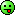As others have noted, it's a deliberately confusing question. They label the boxes. What you need to do is label the balls.There are 6 balls; Box 1 contains balls A and B, both gold. Box 2 contains C and D; C is gold, D is silver. Box 3 contains E and F, both silver.You picked a ball. It's gold. It could be Ball A, B, or C. You don't know which.If it's Ball A, the other ball in the box is gold.If it's Ball B, the other ball in the box is gold.If it's Ball C, the other ball in the box is silver.2 out of 3 possibilities, the other ball is gold.When in doubt, list out all the possible outcomes and count 'em up. 1 Like Re: If You Fail This Test, Then Stop Posting Here by Sammy07: 10:20pm On May 23 falcon01:1/1 and 1/2 = 2/3The guy nah Olodo OLODO As others have noted, it's a deliberately confusing question. They label the boxes. What you need to do is label the balls.There are 6 balls; Box 1 contains balls A and B, both gold. Box 2 contains C and D; C is gold, D is silver. Box 3 contains E and F, both silver.You picked a ball. It's gold. It could be Ball A, B, or C. You don't know which.If it's Ball A, the other ball in the box is gold.If it's Ball B, the other ball in the box is gold.If it's Ball C, the other ball in the box is silver.2 out of 3 possibilities, the other ball is gold.When in doubt, list out all the possible outcomes and count 'em up. Re: If You Fail This Test, Then Stop Posting Here by Sammy07: 10:23pm On May 23 BabaRamota1980:Its 2/5The guy nah Olodo OLODO As others have noted, it's a deliberately confusing question. They label the boxes. What you need to do is label the balls.There are 6 balls; Box 1 contains balls A and B, both gold. Box 2 contains C and D; C is gold, D is silver. Box 3 contains E and F, both silver.You picked a ball. It's gold. It could be Ball A, B, or C. You don't know which.If it's Ball A, the other ball in the box is gold.If it's Ball B, the other ball in the box is gold.If it's Ball C, the other ball in the box is silver.2 out of 3 possibilities, the other ball is gold.When in doubt, list out all the possible outcomes and count 'em up. Re: If You Fail This Test, Then Stop Posting Here by APCLyingBastard: 10:24pm On May 23 Sammy07:The guy nah Olodo OLODO As others have noted, it's a deliberately confusing question. They label the boxes. What you need to do is label the balls.There are 6 balls; Box 1 contains balls A and B, both gold. Box 2 contains C and D; C is gold, D is silver. Box 3 contains E and F, both silver.You picked a ball. It's gold. It could be Ball A, B, or C. You don't know which.If it's Ball A, the other ball in the box is gold.If it's Ball B, the other ball in the box is gold.If it's Ball C, the other ball in the box is silver.2 out of 3 possibilities, the other ball is gold.When in doubt, list out all the possible outcomes and count 'em up.falcon01:1/1 and 1/2 = 2/3Let me put you out of your pathetic ignorance and misery. let me provide the LOGIC behind the answer which is 1/2 or 50%.You have 6 balls , 3 gold and 3 silver arranged in 3 boxes with 2 gold, 2 silver and one of either in separate boxes.You draw a gold ball.The question that was asked is what is the probability of you drawing a second ball from the same box?That means you drew from either the box with 2 gold balls or the one with a single gold and silver ball.Now you have only 3 balls left in two boxes where you had gold ballsThe chances of you having drawn a gold ball from the box with either 2 gold balls or a single gold ball is inconsequential as the main question is the follow-up event of drawing a second ball.Hence, you are left with deciding if your first draw was from either the box with 2 gold balls or the one with a single gold and a single silver ball.The probability that you will pick a gold ball is 50% because if you drew from the first box with 2 gold balls , it would have been 100% but then again you might have drawn from the second box with a single gold ball and a silver ball.So you are either going to pick a Gold ball (if you selected from the first box) or a silver ball (if your first draw was from the second). 6 Likes Re: If You Fail This Test, Then Stop Posting Here by falcon01: 10:27pm On May 23 APCLyingBastard:Let me put you out of your pathetic ignorance and misery. let me provide the LOGIC behind the answer which is 1/2 or 50%.You have 6 balls , 3 gold and 3 silver arranged in 3 boxes with 2 gold, 2 silver and one of either in separate boxes.You draw a gold ball.The question that was asked is what is the probability of you drawing a second ball from the same box?That means you drew from either the box with 2 gold balls or the one with a single gold and silver ball.Now you have only 3 balls left in two boxes where you had gold ballsThe chances of you having drawn a gold ball from the box with either 2 gold balls or a single gold ball is inconsequential as the main question is the follow-up event of drawing a second ball.Hence, you are left with deciding if your first draw was from either the box with 2 gold balls or the one with a single gold and a single silver ball.The probability that you will pick a gold ball is 50% because if you drew from the first box with 2 gold balls , it would have been 100% but then again you might have drawn from the second box with a single gold ball and a silver ball.So you are either going to pick a Gold ball (if you selected from the first box) or a silver ball (if your first draw was from the second).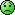haba nw why are you insulting me?, I'm trying my best. Re: If You Fail This Test, Then Stop Posting Here by Sammy07: 10:28pm On May 23 APCLyingBastard:Let me put you out of your pathetic ignorance and misery. let me provide the LOGIC behind the answer which is 1/2 or 50%.You have 6 balls , 3 gold and 3 silver arranged in 3 boxes with 2 gold, 2 silver and one of either in separate boxes.You draw a gold ball.The question that was asked is what is the probability of you drawing a second ball from the same box?That means you drew from either the box with 2 gold balls or the one with a single gold and silver ball.Now you have only 3 balls left in two boxes where you had gold ballsThe chances of you having drawn a gold ball from the box with either 2 gold balls or a single gold ball is inconsequential as the main question is the follow-up event of drawing a second ball.Hence, you are left with deciding if your first draw was from either the box with 2 gold balls or the one with a single gold and a single silver ball.The probability that you will pick a gold ball is 50% because if you drew from the first box with 2 gold balls , it would have been 100% but then again you might have drawn from the second box with a single gold ball and a silver ball.So you are either going to pick a Gold ball (if you selected from the first box) or a silver ball (if your first draw was from the second).Olodo. OLODO Go to school you no gree As others have noted, it's a deliberately confusing question. They label the boxes. What you need to do is label the balls. There are 6 balls; Box 1 contains balls A and B, both gold. Box 2 contains C and D; C is gold, D is silver. Box 3 contains E and F, both silver.You picked a ball. It's gold. It could be Ball A, B, or C. You don't know which. If it's Ball A, the other ball in the box is gold.If it's Ball B, the other ball in the box is gold.If it's Ball C, the other ball in the box is silver.2 out of 3 possibilities, the other ball is gold.When in doubt, list out all the possible outcomes and count 'em up. Re: If You Fail This Test, Then Stop Posting Here by APCLyingBastard: 10:29pm On May 23 Sammy07:Olodo. OLODO Go to school you no gree As others have noted, it's a deliberately confusing question. They label the boxes. What you need to do is label the balls. There are 6 balls; Box 1 contains balls A and B, both gold. Box 2 contains C and D; C is gold, D is silver. Box 3 contains E and F, both silver.You picked a ball. It's gold. It could be Ball A, B, or C. You don't know which. If it's Ball A, the other ball in the box is gold.If it's Ball B, the other ball in the box is gold.If it's Ball C, the other ball in the box is silver.2 out of 3 possibilities, the other ball is gold.When in doubt, list out all the possible outcomes and count 'em up.I see this is how you are reasoning it from pic below.But this is the absolute wrong answer.Why, because you will have to pick from the same box and not another box!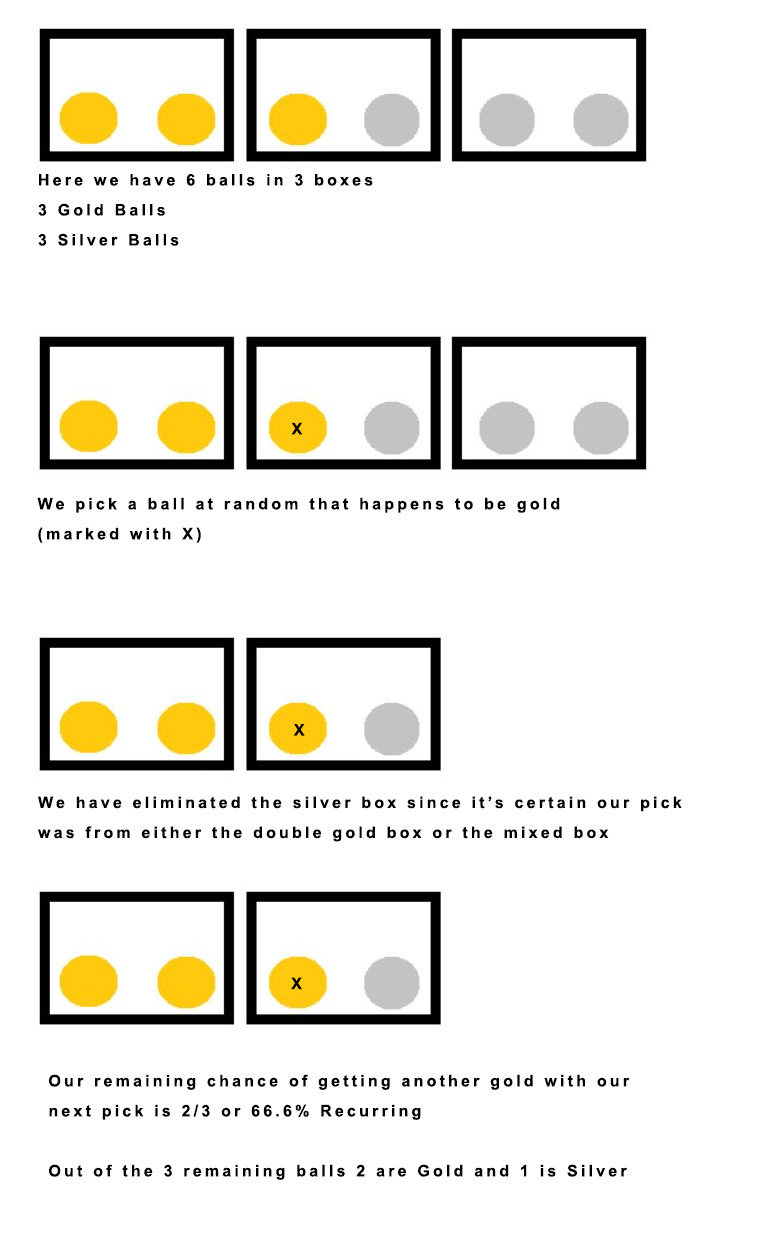Re: If You Fail This Test, Then Stop Posting Here by Sammy07: 10:33pm On May 23 APCLyingBastard:[s][/s]According to your pics, you marked only the second box.Why ?Why wasn't it picked in the 1st box. Olodo. See how I labelled it here with detailed explanations.OLODO As others have noted, it's a deliberately confusing question. They label the boxes. What you need to do is label the balls. There are 6 balls; Box 1 contains balls A and B, both gold. Box 2 contains C and D; C is gold, D is silver. Box 3 contains E and F, both silver.You picked a ball. It's gold. It could be Ball A, B, or C. You don't know which. If it's Ball A, the other ball in the box is gold.If it's Ball B, the other ball in the box is gold.If it's Ball C, the other ball in the box is silver.2 out of 3 possibilities, the other ball is gold.When in doubt, list out all the possible outcomes and count 'em up. Re: If You Fail This Test, Then Stop Posting Here by APCLyingBastard: 10:35pm On May 23 Sammy07:Olodo. OLODO Go to school you no gree As others have noted, it's a deliberately confusing question. They label the boxes. What you need to do is label the balls. There are 6 balls; Box 1 contains balls A and B, both gold. Box 2 contains C and D; C is gold, D is silver. Box 3 contains E and F, both silver.You picked a ball. It's gold. It could be Ball A, B, or C. You don't know which. If it's Ball A, the other ball in the box is gold.If it's Ball B, the other ball in the box is gold.If it's Ball C, the other ball in the box is silver.2 out of 3 possibilities, the other ball is gold.When in doubt, list out all the possible outcomes and count 'em up.When you see that the probability is not in the picking the ball but the box you chose, that is when the answer will hit you.By picking the gold ball, you eliminated the box containing 2 silver balls.You are now left with two boxes.You either drew from the first with two gold or the one with a silver and a gold ball.Forget about the number of balls and think about the box.Here lies the logic.

(1) (2) (3) (4) (5) (6) (Reply)

(Go Up)

 Sections: politics (1) business autos (1) jobs (1) career education (1) romance computers phones travel sports fashion health religion celebs tv-movies music-radio literature webmasters programming techmarket Nairaland - Copyright © 2005 - 2020 Oluwaseun Osewa. All rights reserved. See How To Advertise. 218Disclaimer: Every Nairaland member is solely responsible for anything that he/she posts or uploads on Nairaland.